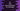# C# program to find the area and perimeter of a rectangle## C# program to find the area and perimeter of a rectangle:

In this post, we will learn how to find the area of a rectangle in C sharp. To find the rectangle area, we need to get the height and width of the rectangle as user input. Then, we can use the below formula to find the area and perimeter of that rectangle.

With this program, you will learn how to take user inputs in C#, how to do mathematical calculations and how to print messages.

### Formula to find the area and perimeter of a rectangle:

If height is the height of the rectangle and width is the width of the rectangle, we can use the below formule to find the area and perimeter:

``````area = height * width
perimeter = 2 * (height + width)``````

### C# program:

Let’s write it down in a C# program:

``````using System;

public class MainClass
{
public static void Main()
{
Console.WriteLine("Enter the width of the rectangle:");

Console.WriteLine("Enter the height of the rectangle:");

double area = height * width;
double perimeter = 2 * (height + width);

Console.WriteLine("Area: {0}", area);
Console.WriteLine("Perimeter: {0}", perimeter);
}
}``````

Here,

• The program first asks the user to enter the width of the rectangle using Console.WriteLine. It then reads the value using Console.ReadLine. But, Console.ReadLine returns the value as String. So, we are parsing it as double using double.Parse method. This value is stored in the width variable.
• Similarly, it reads the height of the rectangle and stores it in height.
• It is finding the area and perimeter of the rectangle by using the same formule I have mentioned above. The calculated values of area and perimeter are stored in the double variable area and perimeter respectively.
• The last two Console.WriteLine lines are used to print the calculated area and perimeter values.

If you run this program, it will print outputs as like below:

``````Enter the width of the rectangle:
10
Enter the height of the rectangle:
20
Area: 200
Perimeter: 60``````

You can use the same program to find the area and perimeter of squares as well.